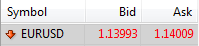Understanding Forex TerminologyUnderstanding Forex Terminology

The aim of this article is to introduce the reader to some of the terminology used in Forex. If one aims to trade forex one has to understand these terms. These concepts will be further used when one has to apply risk management principles. There are a few simple calculations that l will do when explaining a concept.

What is a pip?

Currency price moves are measured in pips. This concept is best explained with an example. Let the exchange rate of the EUR/USD(Euro to Dollar) be 1.14199. In this example 1 pip = 0.0001. A pipette is 0.1 of a Pip thus a pipette is 0.00001.The spread is the difference between the Bid price and the Ask price. The bid is the price at which the market is willing to buy and the ask is the price the market is willing to sell. As an individual, you buy at the asking price and sell at the bid price. If you look at the quote below the spread is 1.6 pips (=1.14009 – 1.13993).What is a Lot?

A lot is the number of units of a currency that you buy. If you own 1 Lot size then for every 1 pip move your account will move up or down by US\$10. If you own a mini lot that is 0.1 of a Lot then for every pip move you lose or gain US\$1.

Transaction Costs

The amount you usually pay for every trade you make is equal to Number of Lots × USD10 × spread in pips. Taking the above quote for the EUR/USD and if we transact 2 Lots the cost of the transaction will be USD32(=2×USD10×1.6).

You may also like...Tinaye is a self taught forex trader. He holds an honours degree in mathematics and is currently pursuing a Financial Risk Management (FRM) qualification.

Top contributors